# Bayes Test for One Proportion

### Home | Academic Articles

Contrast of frequentist vs. Bayesian approach

Suppose we have a salesperson who has the following track record over a 3-week period:

 Number of sales calls Number of sales Week 1 5 1 Week 2 5 0 Week 3 5 5 Total 15 6

If the goal is to have the sales rate exceed 20%, can we conclude at a 5% level of significance that the goal is being achieved?

The null and alternative hypotheses are:

Ho: p < 20%

Ha: p > 20%

From a frequentist point of view, the p-value is the probability of having at least 6 sales in the 15 trials. If p = 20%, P(X > 6) = 1 – P(X < 5) = 1 – 0.939 = 0.061 = 6.1%. Since 6.1% > 5%, we do not reject the null hypothesis and conclude that the goal is not being achieved.

From a Bayesian point of view, the p-value can be viewed as the probability of the null hypothesis being true.

For the prior distribution of p, we can assume that it can uniformly take any value between 0 and 1. Thus, f(p) = 1.

Since we have 6 successes and 9 failures, the posterior distribution of p is proportional to p6(1 – p)9. This indicates that p follows a Beta distribution with α = 7 and β = 10. Thus, when we solve for P(p < 0.2), we get: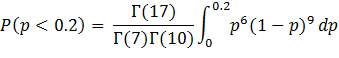Once you work through the math, P(p < 0.2) is equivalent to the probability of having at least 7 successes in 16 trials given p = 0.2. This works out to 0.0267 = 2.67%. Since 2.67% < 5%, we reject the null hypothesis and conclude the goal is being achieved.

Asymptotic results

Suppose the null and alternative hypotheses are changed to:

Ho: p < 40%

Ha: p > 40%

From the frequentist point of view, the p-value = P(X > 6) = 1 – P(X < 5) = 1 – 0.403 = 0.597 = 59.7%. From the Bayesian point of view, the p-value = P(p < 0.4) which is equivalent to the probability of at least 7 successes in 16 trials given p = 0.4. This works out to 0.4728 = 47.28%.

Now, the sample proportion (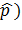= 6/15 = 0.4. For various values of n and p, we want to find the probability of the null hypothesis being true.

 n | p 0.2 0.4 0.5 0.6 0.7 0.8 0.9 15 2.67% 47.28% 77.28% 94.17% 99.29% 99.98% 100% 50 0.04% 48.48% 91.96% 99.78% 100% 100% 100% 100 0 48.92% 97.7% 100% 100% 100% 100% 500 0 49.51% 100% 100% 100% 100% 100% 1000 0 49.66% 100% 100% 100% 100% 100%

For p < 40%, as n increases, P(Ho being true) decreases and eventually reaches a probability of zero for all intents and purposes.

However, if p = 40%, as n increases, P(Ho being true) approaches 50%.

Finally, for p > 50%, as n increases, P(Ho being true) increases and eventually reaches a probability of 100% for all intents and purposes.

These probabilities are roughly comparable to p-values from the frequentist school. For example, if p = 0.2, n = 100 and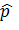= 0.4, the value of the test statistic would be: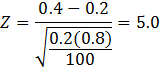The p-value = P(Z > 5) = 0 for all intents and purposes.

If p = 0.4 and= 0.4, Z = 0 regardless of the sample size and P(Z > 0) = 0.5.

Finally, if p = 0.5, n = 100 and= 0.4, the value of the test statistic would be: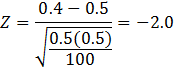The p-value = P(Z > -2) = 97.73%.

Jeffrey’s non-informative prior with test for p

If Jeffrey’s non-informative prior is used, α = x and β = n – x. In the first example with n = 15 and x = 6, the posterior distribution of p is proportional to p5(1 – p)8.

Given the null and alternative hypotheses:

Ho: p < 20%

Ha: p > 20%

P(p < 20%) is equivalent to the probability of having at least 6 successes in 14 trials given p = 0.2. This works out to 0.0439 = 4.39%.

In general, P(Ho being true) = P(p < po) = 1 – P(X < x-1 | n-1, po) in which po represents the hypothesis proportion.

If x = n, P(Ho being true) = 1 – P(X < n-1 | n-1, po) = 1 – 1 = 0.

Thus, Jeffrey’s non-informative prior cannot be used for hypothesis testing. This is also due to the fact that P(p) = 1/[p(1-p)] is not a proper PDF as illustrated here: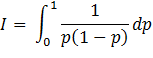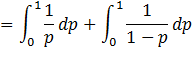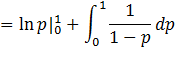Let u = 1 – p. Then du = -dp.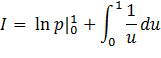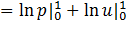The result is undefined since ln(0) is negative infinity.

Reference:

Zellner, Arnold. An Introduction to Bayesian Inference in Econometrics. New York: John Wiley & Sons, 1970.